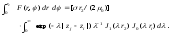#  Preparation of Papers for ieee transactions on medical imaging

 Page 2/7 Date 26.10.2016 Size 148.68 Kb. #466

## MATH

If you are using Word, use either the Microsoft Equation Editor or the MathType add-on (http://www.mathtype.com) for equations in your paper (Insert | Object | Create New | Microsoft Equation or MathType Equation). “Float over text” should not be selected.
1. ## Equations

Number equations consecutively with equation numbers in parentheses flush with the right margin, as in (1). First use the equation editor to create the equation. Then select the “Equation” markup style. Press the tab key and write the equation number in parentheses. To make your equations more compact, you may use the solidus ( / ), the exp function, or appropriate exponents. Use parentheses to avoid ambiguities in denominators. Punctuate equations when they are part of a sentence, as in(1)
Be sure that the symbols in your equation have been defined before the equation appears or immediately following. Italicize symbols (T might refer to temperature, but T is the unit tesla). Refer to “(1),” not “Eq. (1)” or “equation (1),” except at the beginning of a sentence: “Equation (1) is ... .”
1. ## Units

Use either SI (MKS) or CGS as primary units. (SI units are strongly encouraged.) English units may be used as secondary units (in parentheses). For example, write “15 Gb/cm2 (100 Gb/in2).” An exception is when English units are used as identifiers in trade, such as “3½-in disk drive.” Avoid combining SI and CGS units, such as current in amperes and magnetic field in oersteds. This often leads to confusion because equations do not balance dimensionally. If you must use mixed units, clearly state the units for each quantity in an equation.

The SI unit for magnetic field strength H is A/m. However, if you wish to use units of T, either refer to magnetic flux density B or magnetic field strength symbolized as µ0H. Use the center dot to separate compound units, e.g., “A·m2.”

1. ## Some Common Mistakes

The word “data” is plural, not singular. The subscript for the permeability of vacuum µ0 is zero, not a lowercase letter “o.” The term for residual magnetization is “remanence”; the adjective is “remanent”; do not write “remnance” or “remnant.” Use the word “micrometer” instead of “micron.” A graph within a graph is an “inset,” not an “insert.” The word “alternatively” is preferred to the word “alternately” (unless you really mean something that alternates). Use the word “whereas” instead of “while” (unless you are referring to simultaneous events). Do not use the word “essentially” to mean “approximately” or “effectively.” Do not use the word “issue” as a euphemism for “problem.” When compositions are not specified, separate chemical symbols by en-dashes; for example, “NiMn” indicates the intermetallic compound Ni0.5Mn0.5 whereas “Ni–Mn” indicates an alloy of some composition NixMn1-x.

Be aware of the different meanings of the homophones “affect” (usually a verb) and “effect” (usually a noun), “complement” and “compliment,” “discreet” and “discrete,” “principal” (e.g., “principal investigator”) and “principle” (e.g., “principle of measurement”). Do not confuse “imply” and “infer.”

Prefixes such as “non,” “sub,” “micro,” “multi,” and “ultra” are not independent words; they should be joined to the words they modify, usually without a hyphen. There is no period after the “et” in the Latin abbreviation “et al.” (it is also italicized). The abbreviation “i.e.,” means “that is,” and the abbreviation “e.g.,” means “for example” (these abbreviations are not italicized).

A general IEEE styleguide is available at http://www.ieee.org/web/publications/authors/transjnl/index.html